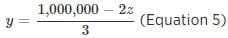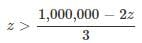A trader purchased three products - Product X...

### Related TestA trader purchased three products - Product X, Product Y, and Product Z - for a sum of \$500,000. Did the trader pay more than \$200,000 for Product Z?
(1) The sum the trader paid for Product X and Product Y combined was 3 times the sum the trader paid for Product X.
(2) The trader paid more to purchase Product Z than to purchase Product Y.
• a)
Statement (1) ALONE is sufficient, but statement (2) alone is not sufficient to answer the question asked;
• b)
Statement (2) ALONE is sufficient, but statement (1) alone is not sufficient to answer the question asked;
• c)
BOTH statements (1) and (2) TOGETHER are sufficient to answer the question asked,but NEITHER statement ALONE is sufficient;
• d)
• e)
Statements (1) and (2) TOGETHER are NOT sufficient to answer the question asked, and additional data are needed.EduRev GMAT
We are given that the amount spent on buying Product X, Product Y, and Product Z is \$500,000.
Say the amounts spent on buying Product X, Product Y, and Product Z are x,y, and z, respectively.
⇒ x + y + z = 500,000 (Equation 1)
We have to determine whether z>200,000.
Statement 1:
We are given that the sum the trader paid for Product X and Product Y combined was 3 times the sum the trader paid for Product X.
⇒ x + y = 3x
y = 2x (Equation 2)
Substituting equation (2) in equation (1):
⇒ x + 2x + z = 500,000
⇒ 3x + z = 500,000
We have an unknown x. So, we cannot determine whether z > 200,000. Insufficient!
Statement 2:
We are given that the trader paid more to purchase Product Z than to purchase Product Y.
⇒ z > y (Inequality 3)
With the help of equation (1): x + y + z = 500,000 and inequality (3): z > y, we cannot determine whether z > 200,000. Insufficient!
Statement 1 & 2 together:
Let's put down the two equations (1) and (2) and the inequality (3).
x + y + z = 500,000 (Equation 1)
y = 2x (Equation 2)
z > y (Inequality 3)
Since equation (3) has only z and y variables, let's eliminate variable x from equation (1) and (2).
From equation (2), we have x = y/2. By plugging in the value x in equation (1), we get:
y/2 + y + z = 500,000
y + 2y + 2z = 1,000,000
3y + 2z = 1,000,000 (Equation 4)Substituting (5) in (3):5z > 1,000,000
z > 200,000
So, the answer is 'Yes'. The two statements together are sufficient!
Alternate approach:
Alternate way of solving further after Equation (4): 3y + 2z = 1,000,000
Let's assume that y = z, and see what are their values. Say y = z = p
Thus, 3p+2p = 1,000,000
⇒5p = 1,000,000
⇒p = 200,000
Thus, y = z = 200,000;
However, this goes against the fact given in inequality (3): z > y.
Thus, y < 200,000 & z > 200,000. The answer is 'Yes'. Sufficient!View courses related to this question Explore GMAT courses
 Explore GMAT coursesView courses related to this question1 Crore+ students have signed up on EduRev. Have you?

• ### Directions: Each Data Sufficiency problem consists of a question and two st... more(Scan QR code)